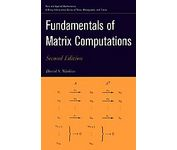# Fundamentals of MATRIX COMPUTATIONS

## by David S. Watkins.Introduction
On this page you will find links to various problems from Dr. Watkins excellent book. This book is a very nice introduction to numerical linear algebra. It is quite enjoyable to read and has a very nice set of problems to work. I have begun to LaTeX solutions to the problems from the second edition and what I have been able to do are presented below. Note currently due to time restrictions I have not been able to LaTeX all that I would have like. As I get more time I will be continually adding solutions. This is a work in progress. While of lesser value, I have also included my scanned solutions to many of the problems from the first edition.

Miscellaneous Matlab Code:
Chapter 1 (Gaussian Elimination and Its Variants):
Matlab Code For Various Problems In the SECOND EDITION:
Chapter 1 (Gaussian Elimination and Its Variants):
• exercise_1_1_9.m (timings of matrix vector multiplication)
• exercise_1_1_10.m (further timings of matrix vector multiplication)
• exercise_1_1_11: (BLAS2 FORTRAN and C version of matrix vector multiplication)
• The BLAS routines provide excellent implementations of this functionality:
• dgemv.f (FORTRAN BLAS2 function dgemv.f)
• dgemv.c (C BLAS2 function dgemv.c)
• exercise_1_1_14.m (timings of matrix multiplication)
• exercise_1_2_7.m (numerical solution for circuit voltages)
• exercise_1_2_9.m (numerical solution for circuit currents)
• exercise_1_2_11.m (numerical solution for the cart displacements)
• exercise_1_3_22 (FORTRAN routines for solving triangular systems A in Ax=b):
• For general purpose use I would recommend the (BLAS routine dtrsv)
• exercise_1_4_23.m (using the Cholesky decomposition to test for positive definiteness)
• exercise_1_6_3.m (visualizing sparse Cholesky factorizations)
• exercise_1_6_4.m (visualizing sparse Cholesky factorizations on the delsq matrix)
• exercise_1_6_5.m (visualizing sparse Cholesky factorizations on the airfoil matrix)
• exercise_1_6_6.m (visualizing various unknown orderings on a finite element matrix (wathen))
• exercise_1_8_10.m (simple experiments with Matlab lu command)
• condif.m (generates the coefficient matrix for a discretization of the 1d convection-diffusion equation)
• exercise_1_8_22 (routines for performing Gaussian elimination with partial pivoting):
• For pedagogical purposes, direct implementations of the solution routines from the book are given below
Chapter 2 (Sensitivity of Linear Systems):
Chapter 7 (Iterative Methods for Linear Systems):
• The problems above may require the core numeric algorithms below
• jacobi.m (An implemenation of the Jacobi iterative method to solve Ax=b)
• gseidel.m (An implementation of the Gauss-Seidel iterative method to solve Ax=b)
• sor.m (An implementation of the SOR iterative method to solve Ax=b)

John Weatherwax